# PHYS-GA-2023 HW3 - P5 - Marek Narozniak¶

Problems 1-4 have been submitted in a form of PDF document. This notebook is submission of problem 5.

To complete this homework I am going to be using following dependencies.

In :
import numpy as np

import qiskit
from qiskit.tools.visualization import plot_histogram
from qiskit import QuantumCircuit, ClassicalRegister, QuantumRegister
from qiskit import Aer, execute

import itertools
backend = qiskit.BasicAer.get_backend('qasm_simulator')

import matplotlib.pyplot as plt
%matplotlib inline


A superdense coding circuit consists of two qubits, but let us setup a function creating $N$ quantum registers and $N$ classical registers.

In :
def makeCircuit(N):
q = QuantumRegister(2)
c = ClassicalRegister(2)
qc = QuantumCircuit(q, c)
return q, c, qc


And a function that builds superdense coding circuit and its diagram.

In :
def superDenseCoding(b1, b2, shots=1024):
q, c, qc = makeCircuit(2)
# prepare share entangled state
qc.h(q)
qc.cx(q, q)
# superdense coding operation depend on sended binary bits
# this part of the circuit is classically controlled
if b1: qc.x(q)
if b2: qc.z(q)
# suppose q0 register is sent to receiver
# decode the transfered information by the receiver
qc.cx(q, q)
qc.h(q)
# measurement
qc.measure(q, c)
# build diagram for visualisation
diagram = qc.draw(output="mpl")
# perform simulation and extract counts
job = qiskit.execute(qc, backend, shots=shots)
result = job.result()
counts = result.get_counts()
comb = ["".join(seq) for seq in itertools.product("01", repeat=2)]
for key in comb:
if key not in counts.keys():
counts[key] = 0
# return everything
return qc, diagram, counts


## Testing¶

Now we are going to perform a test for all the outcomes - we will send all four possible combinations of two classical bits from Alice to Bob by transfering a single quantum register $q_0$.

### Sending 00¶

Build and simulate the circuit that transfers $00$ to Bob.

In :
qc, diagram, counts = superDenseCoding(0, 0)


Visualize the circuit diagram.

In :
diagram

Out: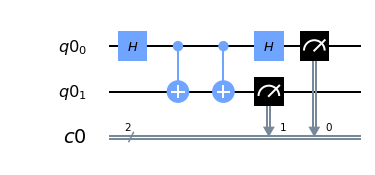Plot the histogram of counts for all measured outcomes on Bob's side.

In :
plot_histogram(counts)

Out: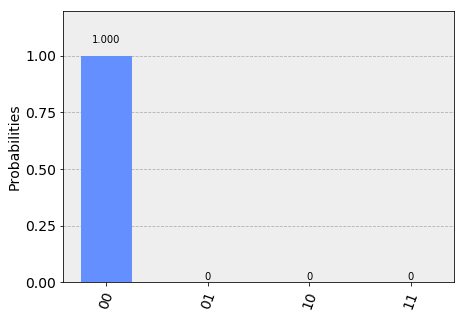As we can see, $100$% of times Bob measured configuration $00$, so $00$ was successfully transfered from Alice to Bob.

### Sending 01¶

Build and simulate the circuit that transfers $01$ to Bob.

In :
qc, diagram, counts = superDenseCoding(0, 1)


Visualize the circuit diagram.

In :
diagram

Out: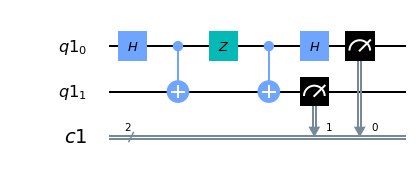Plot the histogram of counts for all measured outcomes on Bob's side.

In :
plot_histogram(counts)

Out: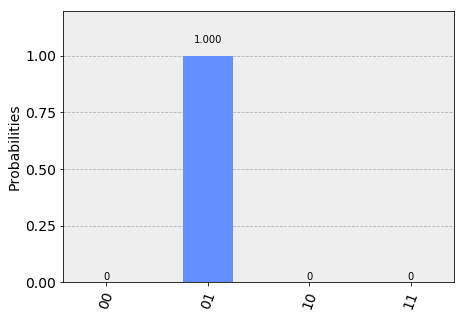As we can see, $100$% of times Bob measured configuration $01$, so $01$ was successfully transfered from Alice to Bob.

### Sending 10¶

Build and simulate the circuit that transfers $10$ to Bob.

In :
qc, diagram, counts = superDenseCoding(1, 0)


Visualize the circuit diagram.

In :
diagram

Out: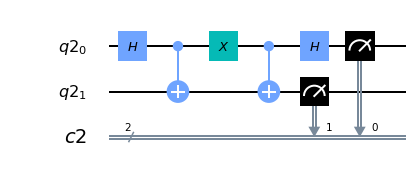Plot the histogram of counts for all measured outcomes on Bob's side.

In :
plot_histogram(counts)

Out: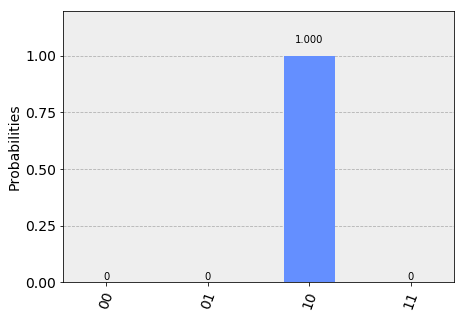As we can see, $100$% of times Bob measured configuration $10$, so $10$ was successfully transfered from Alice to Bob.

### Sending 11¶

Build and simulate the circuit that transfers $11$ to Bob.

In :
qc, diagram, counts = superDenseCoding(1, 1)


Visualize the circuit diagram.

In :
diagram

Out: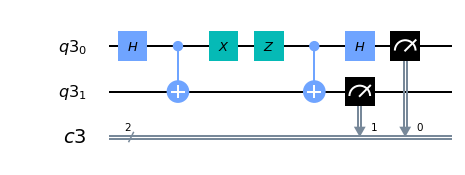Plot the histogram of counts for all measured outcomes on Bob's side.

In :
plot_histogram(counts)

Out: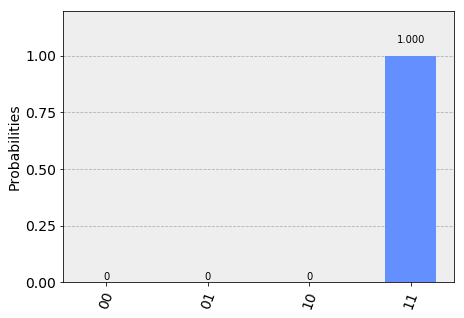As we can see, $100$% of times Bob measured configuration $11$, so $11$ was successfully transfered from Alice to Bob.

## Conclusion¶

We build a function that dynamically constructs and simulates the quantum circuit corresponding to superdense coding. Circuit is dynamically build based on the kind of $2$-bit binary string Alice wants to send to Bob, conceptually Alice would run this part of the function, send single qubit to Bob who would then perform a measurement and receive $2$-bits of information.

We plotted the histograms of measured counts by Bob for each of possible outcomes and it confirmed that he received exactly the bits that Alice intended to send.